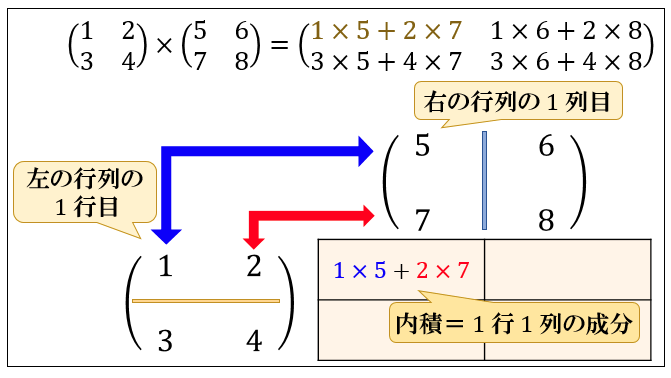# Python Math Series ② Matrix Multiplication

## Background

I'm reading Deep Learning Book, but I get sleepy when I just read the characters, so I'll try to deepen my understanding by implementing the items that appear in python. I don't know if there is demand, but it's in a series (laughs). The table of contents is here. This time I'm going to do matrix multiplication. Since python has abundant libraries, you can calculate without thinking if you rely on it, but I dare to make it myself. *** This is my last article on the ad event calendar. @ Bigface00 will close the end of the calendar. *** ***

## Matrix multiplication

Matrix multiplication can be calculated with the image below. Reference source## Easy implementation

If you refer to the article here, it seems that you can easily implement it by using numpy.dot or numpy.matmal.

``````arr1 = np.arange(4).reshape((2, 2))
print(arr1)
# [[0 1]
#  [2 3]]

arr2 = np.arange(6).reshape((2, 3))
print(arr2)
# [[0 1 2]
#  [3 4 5]]

print(np.dot(arr1, arr2))
# [[ 3  4  5]
#  [ 9 14 19]]

print(arr1.dot(arr2))
# [[ 3  4  5]
#  [ 9 14 19]]

print(np.matmul(arr1, arr2))
# [[ 3  4  5]
#  [ 9 14 19]]
``````

First, count the number of rows and columns of the final answer. Since the number of rows of the answer is the number of rows of the previous matrix and the number of columns of the answer is the number of columns of the latter matrix, the for statement is rotated based on that. In the third for statement, we are calculating the rows of the previous matrix and the columns of the subsequent matrix. I put the calculation result for each column of the previous matrix in tmp_list, and finally put all the calculation results in ans_list.

``````def matrix_mlp(list1, list2):
ans_row = len(list1)
ans_col = len(list2)
ans_list = []
for i in range(ans_row):
tmp_list = []
for j in range(ans_col):
tmp = 0
for k in range(ans_row):
tmp += list1[i][k] * list2[k][j]
tmp_list.append(tmp)
ans_list.append(tmp_list)
return ans_list

def main():
print("~~matrix_multiple_test~~")
arr1 = np.arange(4).reshape((2, 2))
arr2 = np.arange(6).reshape((2, 3))
print("Before1  :", arr1.tolist())
print("Before2  :", arr2.tolist())
print("correct  :", np.dot(arr1, arr2).tolist())